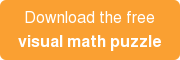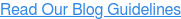### Blog: Subscribe NowFeatured Podcast

Listen Now

# Getting Curious About Geometry Through Visual Math Puzzles [Activity]

When learning about math in school, I, like most young students, thought math was about numbers and symbols, things like +, -, 12, AB and X (and when I got to highschool: Δ, ∑, and of course, the classic ∫).

It wasn’t until I was an undergraduate in college that I learned that math was more than doing computations, math is also about exploring logical structures and asking questions. While symbols are important, they are not the only way you can “do math.” Instead of pushing symbols around, try visualizing the thing you are thinking about. Visuals have the power to highlight structures that can be hidden between the lines symbolically. Many mathematicians work visually, because it makes their work easier, or because they have to. To quote mathematician William P Thurston:

People have very powerful facilities for taking in information visually or kinesthetically, and thinking with their spatial sense. On the other hand, they do not have a very good built-in facility for inverse vision, that is, turning an internal spatial understanding back into a two-dimensional image. Consequently, mathematicians usually have fewer and poorer figures in their papers and books than in their heads.

Here’s an example of visual problem solving you can try on your own and with geometry students:

Puzzle: How many pennies does it take to surround the center penny?

This math puzzle allows student to apply ideas found in the following common core standards:
Lines, Line Segments [CCSS.MATH.CONTENT.4.G.A.1]
Equilateral Triangles [CCSS.MATH.CONTENT.5.G.B.3]
Composition Idea [CCSS.MATH.CONTENT.6.G.A.1]TRY IT: Surround one penny with the other pennies, so that each penny touches the center penny.
DISCUSSION:What do we know about circles?

• They have a center.
• They have a radius, which is a line from the center to the outside of the circle. The radius is R units long.
• Everything that is “radius” distance away from the center is a point on the circle.
• If you can touch a spot in the circle with the radius, then it is inside the circle.
• All the angles around the center need to add up to 360.TRY IT: Put two pennies next to each other so that they touch.
DISCUSSION: What can we say about two circles that are touching?

• If they are touching, then the distance between the centersis 2 times the radius.
• They touch at one point
• That point looks like the midpoint of the line between the centers. Can you explain why that might be?TRY IT: Add a penny so that it touches both pennies.

DISCUSSION: What can we say about three circles touching?
• We can see that the distance between any two centers is two times the radius.
• The centers form a triangle!What do we know about triangles?

• When all 3 sides are equal, the triangle is called an equilateral triangle, which has certain properties:
• All the sides are the same and all the angles are the same.
• All the angles in a triangle add up to 180.
• If all the angles are the same, and they all add to 180, then each angle must be 60 degreesWhat do you think will happen if we keep adding circles?

TRY IT: Keep adding pennies until the center penny is surrounded again.
DISCUSSION: What shape do the triangles between the pennies create?
What do we know about hexagons?

• Each tip of the triangle is 60 degrees.
• When you fit six of them together, all six of them add up to 360 degrees, so there are no gaps.FURTHER DISCUSSION:
Does this work with all coins?
If we changed the size of the coins on the inside to be a quarter, does that still work?
Are there coins that leave gaps?This puzzle would have been hard to explore had we not played with a physical visualization, or drawn it on paper.

Visuals have the ability to uncover things that are hidden in the words, and give you that much more power when solving problems. Even though mathematicians have to go back and prove things formally with symbols, they often get the ideas for their proofs from visualizations, mental or otherwise. Visualizations are worth 1,000 symbols when it comes to understanding how all the pieces come together.

This is not the only way to solve this problem, nor even the only way to visualise it. Encourage students to explore in their own ways.Further Math Questions and Resources:
This puzzle is really one of just many in the sphere of “Circle Packing” questions. Take a look at Wikipedia as a starting point for more questions, in particular, look at the triangular and hexagonal packings. Look familiar? Be careful not to lose too much time following links!